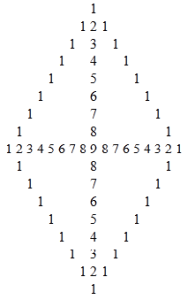# How to make a numeric polygon shows only one and a plus sign with numbers.

We get the output of a numeric polygon using php ‘Loops’. We apply the ‘Loops’ with logic in coding to get the diamond patterns in php.
Here we use eight ‘Loops’. First and fifth are main and other are nested in these.First ‘Loop’ is used for number of lines in upper triangle and fifth loop is used for number of lines in lower triangle.
Second ‘Loop’ is used to print spaces, third ‘Loop’ is used for left side right angle triangle that will show counting according line number and fourth for loop is used for right side right angle triangle that will show reverse counting according to last line number.
Sixth for ‘Loop’ is used to print spaces and seventh is used to show reverse counting in decreasing order in lower left triangle(right angle triangle) and eighth is used to show counting in reverse order in right side triangle(right angle triangle).
After get a polygon we apply if-else in loop conditions and this will show only ‘1’ on its border and make a plus sign among all the polygon numbers. Here \$n is used for number of lines in first loop and number of spaces in second loop.

 ```\$m=1; \$n=9; \$z=9; for(\$i=1; \$i<=\$n; \$i++){ for(\$j=\$i; \$j<=\$n-1; \$j++){ echo "   "; } for(\$k=1; \$k<=\$m; \$k++){ if(\$k==\$m || \$k==1 || \$i==\$n){ echo \$k ." "; } else{ echo "   "; } } for(\$c=\$m; \$c>1; \$c--){ if(\$c-1 == 1 || \$m==\$n){ echo \$c-1 ." "; } else{ echo "   "; } } echo "
"; \$m++; } for(\$i=1; \$i<=\$n; \$i++){ for(\$j=1; \$j<=\$i; \$j++){ echo "   "; } for(\$k=1; \$k<\$z; \$k++){ if(\$k==1 || \$k==\$z-1){ echo \$k ." "; } else{ echo "   "; } } for(\$c=\$z-2; \$c>=1; \$c--){ if(\$c==1){ echo \$c ." "; } else{ echo "   "; } } echo "
"; \$z--; } ```One Comment
1.